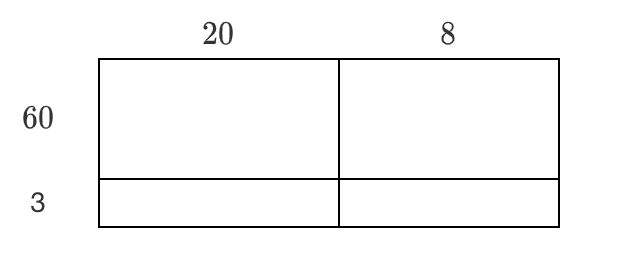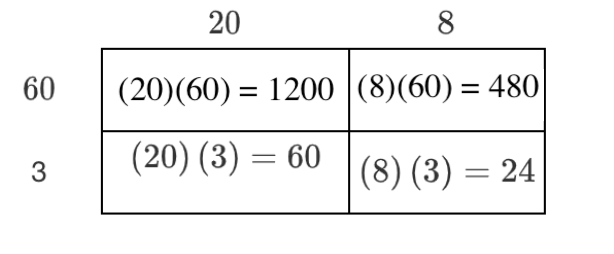### Home > CC1MN > Chapter 3 > Lesson 3.1.5 > Problem3-77

3-77.

Use the Distributive Property to rewrite each product below. Simplify your answer.

1. $28 · 63$

• Write the above product as $(20 + 8)(60 + 3)$ and make a generic rectangle like the one at right. Generic rectangle: Top edge labeled: 20 on left, and + 8 on right. Left edge labeled: 60 on top, and + 3 on bottom. Interior sections are blank.

• Find the product for each part of the rectangle. Added to the Generic rectangle: Interior sections labeled as follows: top left: 20 times 60 = 1200; top right: 8 times 60 = 480. bottom left: 20 times 3 = 60. bottom right: 8 times 3 = 24.

Sum the products: $1200 + 480 + 60 + 24$

$1764$1. $17(59)$

Write this product as $(10 + 7)(50 + 9)$ and create a generic rectangle similar to the one for part (a).

2. $458(15)$

You can use a generic rectangle like the one below to solve this problem.

 Generic Rectangle Top Edge Left is $400$ Top Edge Middle is $50$ Top Edge Right is$8$ Left Edge Top is$10$ Interior top left is blank   $\\$$\quad \\$ Interior top middle is blank$\quad \\$ Interior top right Is blank Left Edge Bottom is $5$ Interior bottom left is blank Interior bottom middle is blank Interior bottom right is blank